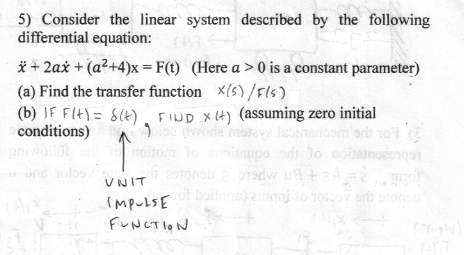Home / Expert Answers / Mechanical Engineering / 5-consider-the-linear-system-described-by-the-following-differential-equation-x-2ax-a2-4-x-pa776

# (Solved): 5) Consider the linear system described by the following differential equation: x+2ax+(a2+4)x= ...5) Consider the linear system described by the following differential equation: (Here is a constant parameter) (a) Find the transfer function (b) (assuming zero initial conditions) VNIT IMPULSE FUNCTION

We have an Answer from Expert

Solution

(a) To find the transfer function, we'll take the Laplace transform of the given differential equation.

Let's denote the Laplace transform variable as 's'.

Taking the Laplace transform of the equation, we get:

We have an Answer from Expert